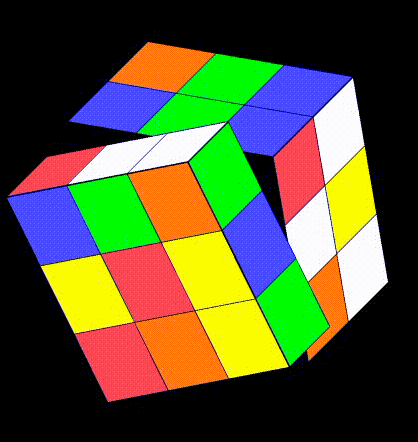{{ message }}

# miskcoo / rubik-cube

Rubik's Cube solver implemented by C++ and OpenGL using Krof/Kociemba algorithm.

## Files

Failed to load latest commit information.
Type
Name
Commit time

# Rubik's Cube Solver

A Rubik's Cube solver implemented by C++ and OpenGL. You can use your mouse to rotate the cube.

See HERE(in Chinese) for more details about the algorithm.## Requirement

• C++11 support (especially the multi-thread library)
• OpenGL
• GLFW

## Usage

`solver -tra`
• -t maximum thread number used to calculate, the default value is 1.
• -r random twist times to generate a cube.
• When using Krof algorithm, the default value is 15.
• When using Krociemba algorithm, the default value is 200.
• -a specify which algorithm will be used to solve the cube.
• Only two algorithms are available: krof, kociemba.
• The default algorithm is kociemba.

Rubik's Cube solver implemented by C++ and OpenGL using Krof/Kociemba algorithm.